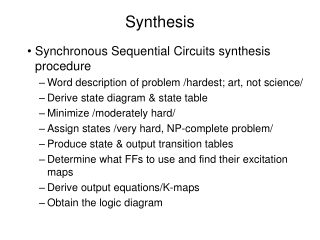DownloadDownload PresentationSynthesis

# Synthesis

Télécharger la présentation## Synthesis

- - - - - - - - - - - - - - - - - - - - - - - - - - - E N D - - - - - - - - - - - - - - - - - - - - - - - - - - -
##### Presentation Transcript

1. Synthesis • Synchronous Sequential Circuits synthesis procedure • Word description of problem /hardest; art, not science/ • Derive state diagram & state table • Minimize /moderately hard/ • Assign states /very hard, NP-complete problem/ • Produce state & output transition tables • Determine what FFs to use and find their excitation maps • Derive output equations/K-maps • Obtain the logic diagram

2. Synthesis • Types of Sequential Circuits • Completely/incompletely specified

3. Synthesis • Example: • Find D FF realization of circuit defined in table (a) • (b): state assignment • (c): transition table • (d): output K-map • (e): excitation K-map

4. Synthesis • Example solution: • Logic diagram

5. Synthesis • Q(t): state of FF when clock signal is activated • Q(t+): state of FF after clock signal has been activated

6. Synthesis • Example is same as before, but use JK FFs • (a): transition table; (b): Excitation tables; (c): Excitation maps

7. Synthesis • Example JK FF solution: • Logic diagram

8. Synthesis Example

9. Synthesis Example

10. Synthesis Example • 01 recognizer timing diagram

11. Synthesis Example

12. Synthesis Example • State table, transition table and output map

13. Synthesis Example • K-maps for a clocked SR realization

14. Synthesis Example

15. Synthesis Example • Feedback for overlapping sequences • Complete state diagram • State table • Reduced state table • State diagram

16. Synthesis Example

17. Synthesis Example • Candy machine state diagram